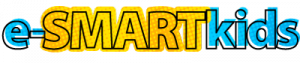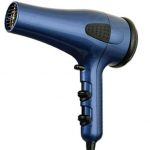# What’s a Watt? Activity

The electricity we use in our homes and schools is measured in units called watts. Because power plants generate so much electricity, the electricity they make is measured in much larger units called megawatts. What’s a megawatt? It’s one million watts. What’s a watt? Well, that’s a little more difficult to explain.

To understand watts you must first understand amps and volts. Amps are a measure of the amount of electricity used. Volts measure the pressure, or force, of electricity. The amps multiplied by the volts gives you the watts, a measure of the work that electricity does per second.Use the formula amps x volts = watts to figure out how many watts these typical appliances use. (Most homes are served by 120-volt electricity, so we will use that as the value for volts in our equation.)

Appliance Amps x Volts = Watts
Hair dryer on high 12 x 120 = ________________
Space heater 10 x 120 = ________________
Vacuum cleaner 8 x 120 = ________________
Ceiling lamp 1 x 120 = ________________
Answering machine .5 x 120 = ________________